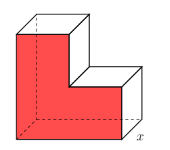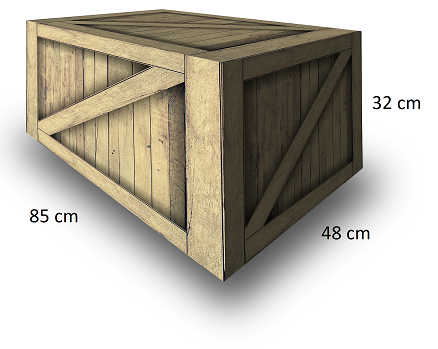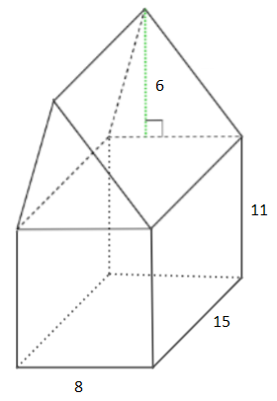Home Practice
For learners and parents For teachers and schools
Textbooks
Full catalogue
Pricing SupportLog in

We think you are located in United States. Is this correct?

# Solving problems involving surface area, volume and capacity

## Worked Example 17.15: Calculating the height of a prism

The volume of the prism is $$4\ 032\text{ cm}^3$$. The area of the shaded region is $$224 \text{ cm}^2$$. Calculate the value of the length, $$x$$.### Use the general formula for volume to find height of prism.

$\text{Volume of prism} = \text{area of base} \times h$

We know the volume of the prism, and we are given the area of the base of the prism, so we can solve for the height, $$x$$:

\begin{align} 4\ 032 &= 224 \times x \\ \frac{4\ 032}{224} &= x \\ 18 &= x \end{align}

The height of the prism $$x = 18 \text{ cm}$$.

## Worked Example 17.16: Calculating the surface area of a rectangular prism

Dintle wants to paint a wooden crate. If she uses $$\text{0,25} \text{ ml}$$ of paint per square centimetre, calculate how much paint she will need to paint the crate. Assume that Dintle will paint every outside surface of the crate. Express your answer in litres.### Find the surface area of the crate.

\begin{align} \text{Surface area of crate} &= 2(\text{area of sides}) + 2(\text{area of ends}) + 2(\text{area of top/bottom}) \\ &= 2(85 \times 32) + 2(48 \times 32) + 2(85 \times 48) \\ &= 2(2\ 720) + 2(1\ 536) + 2(4\ 080) \\ &= 5\ 440 + 3\ 072 + 8\ 160 \\ &= 16\ 672 \end{align}

The surface area of the crate is $$16\ 672 \text{ cm}^2$$.

### Calculate the volume of paint needed.

Dintle will use $$\text{0,25} \text{ ml}$$ of paint per square centimetre.

So, we can calculate:

\begin{align} \text{Volume of paint} &= \text{0,25} \times \text{16 672} \\ &= 4\ 168 \end{align}

Volume of paint needed is $$\text{4 168} \text{ ml}$$.

\begin{align} \text{4 168} \text{ ml} &= \frac{\text{4 168}}{\text{1 000}} \\ &= \text{4,2} \text{ litres} \end{align}

Dintle will need $$\text{4,2} \text{ litres}$$ of paint.

## Worked Example 17.17: Calculating the volume of a complex solid

Calculate the volume of the solid. All measurements are in centimetres.### Find the volume of the cuboid.

\begin{align} \text{Volume of cuboid} &= l \times b \times h \\ &= 8 \times 15 \times 11 \\ &= 1\ 320 \end{align}

### Calculate the volume of the triangular prism.

\begin{align} \text{Volume of triangular prism} &= \frac{1}{2}(b \times h) \\ &= \frac{1}{2}(8 \times 6) \\ &= 24 \end{align}

\begin{align} \text{Volume of solid} &= \text{Volume of cuboid} + \text{Volume of the triangular prism} \\ &= 1\ 320 + 24 \\ &= 1\ 344 \text{ cm}^3 \end{align}